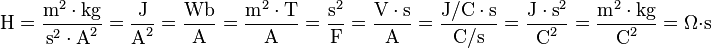# henry (unit) facts for kids

Kids Encyclopedia Facts

In physics, and electronics, the henry (symbol H) is the SI unit of inductance. It is named after Joseph Henry (1797–1878), the American scientist who discovered electromagnetic induction. (In England, Michael Faraday (1791–1867) discovered the same thing independently. They discovered it at about the same time.) The magnetic permeability of a complete vacuum is 4π×10−7 H/m (henry per meter).

The National Institute of Standards and Technology provides guidance for American users of SI to write the plural as henries. People do not capitalize unit names.

## Definition

If the rate of change of current in a circuit is one ampere per second and the resulting electromotive force is one volt, then the inductance of the circuit is one henry. Other equivalent combinations of SI units are as follows:$\mbox{H} = \dfrac{\mbox{m}^2 \cdot \mbox{kg}}{\mbox{s}^{2} \cdot \mbox{A}^2} = \dfrac{\mbox{J}}{\mbox{A}^2} = \dfrac{\mbox{Wb}}{\mbox{A}} = \dfrac{\mbox{m}^2 \cdot \mbox{T}}{\mbox{A}} = \dfrac{\mbox{s}^2}{\mbox{F}} = \dfrac{\mbox{V} \cdot \mbox{s}}{\mbox{A}} = \dfrac{\mbox{J/C} \cdot \mbox{s}}{\mbox{C/s}} = \dfrac{\mbox{J} \cdot \mbox{s}^2}{\mbox{C}^2} = \dfrac{\mbox{m}^2 \cdot \mbox{kg}}{\mbox{C}^2} = \Omega \cdot \mbox{s}$

where

A = ampere
C = coulomb